## DYNAMICS OF EARTH SYSTEMS

Dave Bice
Department of Geosciences
Penn Sate University
University Park, PA 16802
dbice@geosc.psu.edu

Note: This is a shortened version -- a more thorough introduction to modeling systems with STELLA is also available. What follows assumes that you have a copy of STELLA running on your computer, along with the manual, which is very helpful and worth studying.

Some key concepts:

Introduction

One of the most exciting recent developments in the earth sciences has been the move towards looking at the Earth as a large, complex, interconnected system, trying to understand how the system works. This field of study, earth system science, is exciting not just because it is new (in fact, it is not that new -- see the appendix of Strahler and Strahler, 1989), but because it deals with relevant issues such as global climate change and also because it is highly integrative. In conversations with undergraduates at my college, societal relevance and integration of different disciplines are, not surprisingly, some of the things they are constantly looking for in their education. For these and other reasons, earth system science is an attractive addition to many undergraduate earth science courses.

But how does one go about teaching this material? Regardless of how you choose to incorporate earth system science into geology classes, you face some challenges associated with reviewing the relevant physics, chemistry, biology, and meteorology that are needed to understand global systems such as the carbon cycle. Another challenge arises when you search for ways to go beyond the passive description of how various earth systems are constructed and how they operate. Although dynamic systems surround us, we are not generally very good at understanding and predicting their behavior without the aid of some type of model that allows experimentation and observation. In this paper and a companion paper, I describe -- in the form of several examples -- how computer models can provide an effective means for enabling students to understand and explore the dynamics of earth systems. This amounts to developing the systems part of earth system science.

The task of using computer models to explore the dynamics of earth systems is made much easier through the use of a program called STELLA (High Performance Systems, Inc., http://www.hps-inc.com/) STELLA is essentially a graphical programming environment that is specifically designed for modeling systems that can be described by a series of differential equations. Users construct graphical representations of systems and then enter values and expressions corresponding to the components of the system. The program uses this information to construct a set of differential equations that are integrated over time used standard numerical techniques. STELLA runs on both Macs and PCs.

It is important to note that although there is great value in developing the programming skills and facility with differential equations needed to solve these problems without the aid of a program like STELLA, it is nevertheless possible to learn some important things about the dynamics of earth systems without writing your own code. In fact, the algorithms used in STELLA are the same used by most programmers to carry out numerical integration of differential equations.

A First Modeling Exercise: The Water Tub

In order to illustrate some fundamental aspects of modeling and the behavior of dynamic systems, I start with the simplest system imaginable -- a tub of water with a faucet and drain.

1. From the Real World to the Computer Model

The first step in modeling is to define and consider the system as it actually exists in the real world. This involves identifying the components of the system, what material or entity is moving through the system, what processes are involved moving this material or entity, and what kinds of things these processes depend on. I find that this step is often facilitated by drawing a cartoon of the system, as shown in Figure 1.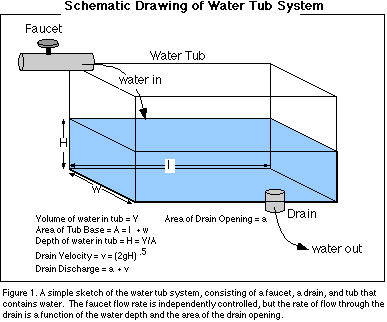The faucet can be adjusted to supply water at whatever rate of flow we choose. In contrast, the rate of flow through the drain is going to be a function of the size of the drain opening and how much water is in the tub because the weight of the water overlying the drain determines the amount of pressure that is forcing the water through the drain. So, the outflow is dependent on the amount of water in the tub. A more precise description of this dependence is provided by Torricelli's Law, which states that the velocity of water flowing out of a drain is equal to the square root of two times the gravitational acceleration times the depth of the water above the drain. The velocity is multiplied by the area of the drain opening to give an outflow in volume of water per unit of time.

Figure 2 shows how this system is represented in STELLA; a box called a reservoir represents the water tub, and two pipes called flows that represent the faucet and the drain -- note that the flows have arrows on one side indicating the direction of material transfer. The circles attached to the flows represent valves that control the flow through the faucet and drain. Another feature of this system are the thin arcuate arrows (connectors) that represent some form of dependence or link between different parts of the system -- for instance, the magnitude of the drain flow is dependent on the area of the drain opening and the velocity of water flowing out the drain, which in turn is dependent on the height of water in the tub, which in turn is dependent on the amount of water in the tub. Below the reservoir are a set of circles called converters that contain information on the details of the water tub and drain opening -- some of these are simple constants, but those with connectors leading to them are variables. Also note that the two flows have cloud symbols at the ends away from the tub, indicating that this is an open system, drawing water from some unspecified source, and sending it to an unspecified sink at the other end.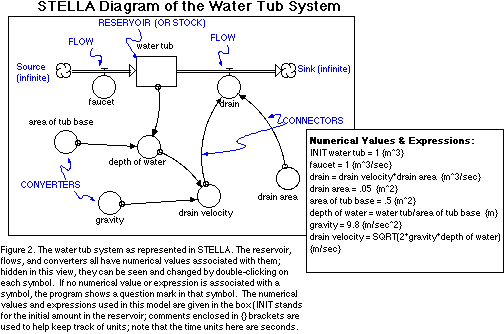The model depicted in Figure 2 represents a complete model that is ready to run after we specify the length of time (the time step, or dt of the calculations) of the simulation and the increments of time over which the program does the calculations. The user can also specify different integration techniques, with the default being Euler's method. The time step is critically important, and before any model results can be seriously considered, it is important to try varying the time step to see how the model results change -- if the results do not change significantly, then the time step is at an acceptable value; otherwise, the time step must be reduced to smaller values.

2. Using the model to illustrate systems concepts

There are a number of important concepts connected to dynamic systems that can easily be illustrated with this simple model.

If we run the model for 100 seconds (Figure 3), we see some interesting changes take place as the system evolves towards what is called a steady state, or a dynamic equilibrium, where the amount in a reservoir stays constant because the inflow and outflow are equal – in this condition, things are changing and water is flowing, but the system as a whole is holding steady. The inflow stays constant over time, but the outflow undergoes an exponential change, increasing until it approaches the same value as the inflow. Initially, the inflow is greater than the outflow, and so the amount of water, and therefore the depth of the water in the tub increase, thus increasing the drain velocity and the outflow; this continues until the inflow and outflow match, at which point water continues to move through the system, but the amount of water in the tub remains the same.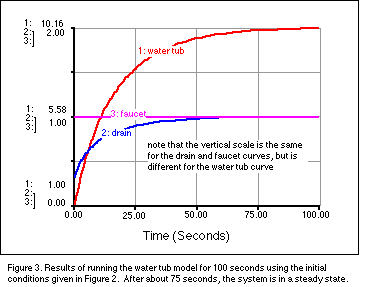When the system is in the steady state, we can define another concept -- the residence time. The residence time is effectively the average length of time that an entity, in this case a water molecule, remains in a reservoir. It is really only meaningful for a reservoir that is at or near a steady state condition. By definition, the residence time is the amount of material in the reservoir, divided by either the inflow or the outflow (they are equal when the reservoir is at steady state). If there are multiple inflows or outflows, then we use the sum of the outflows or inflows to determine the residence time. For our water tub system here, the residence time is thus 10.16 m3 (the amount in the reservoir at the steady state condition) divided by 1 m3/sec per second (the outflow at steady state), giving us 10.16 seconds for the residence time. It is fairly easy to see that if we increase the flow rates, the water moves through the reservoir faster, so the residence time decreases. It is possible then, to calculate any of the above three parameters (residence time, reservoir amount, and inflow or outflow) if the other two are known and if we assume the system is in a steady state. For instance, if we assume that the human population is in a steady state (wishful thinking), and if we know the average residence time, also known as the life span, we can calculate the number of births and deaths in a year. The population is close to 5.6 billion, so if we assume an average life span of 70 years, then we can say that 80 million people are born each year and 80 million people die each year, assuming a steady state. Residence time is an important concept in problems of pollutants in ground water or surface water reservoirs, and also in understanding the long-term effects of greenhouse gases added to the atmosphere.

A closely related concept is that of the response time of a system, which measures how quickly a system recovers and returns to its steady state after some perturbation. We can illustrate this concept by running several simulations, where we vary the starting amount of water in the reservoir and pay attention to how quickly the system gets to its steady state. The results are shown in Figure 4.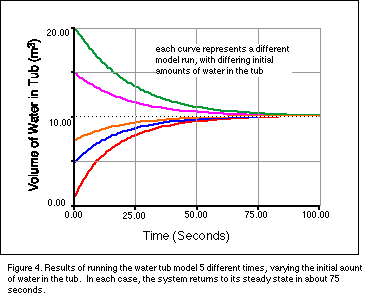It may come as somewhat of a surprise to see that regardless of how great the initial departure from the ending steady state, this system gets to the steady state at about the same time. In an even simpler system, where the outflow (D) is defined as a simple fraction (k) of the amount in the reservoir (W) such that at each instant in time, D = kW, the response time is defined as 1/k -- this turns out to be the time needed for the system to accomplish 63% of the return to its steady state. Also note that in a very simple system, the residence time and the response time are numerically the same even though they are conceptually different. The response time is a very useful concept because if it is known (or hypothesized), we can make some predictions about how quickly the system will respond to a change in a reservoir. Alternatively, if we change one of the inflow or outflow processes, we can predict how that will affect the response time of the system.

A more detailed discussion of the concepts and mathematical formulation of residence time and response time can be found in Rodhe (1992). Alternatively, follow this link.

Another important observation to be made here is that the system evolves to the same steady state in each case, so the steady state of a system (along with the response time) is primarily determined by the nature of the inflows and outflows.

This particular system we've been experimenting with returns a steady state because it contains a negative feedback mechanism in the connection between the drain flow rate and the amount of water in the tub. A negative feedback mechanism is a controlling mechanism, one that tends to counteract or diminish some kind of initial imbalance or perturbation. A good example of another negative feedback mechanism is a simple thermostat in a home that responds to changes from the steady state, returning the home to a specified temperature. Note that the word negative, as used here, does not mean that this is bad feedback; it just means that this feedback mechanism acts to reverse the change that set the feedback mechanism into operation. So if our tub is in its steady state, knocking the system out of its steady state by suddenly dumping in more water will cause a response -- the drain will increase its flow rate, thus decreasing the amount of water in the tub, bringing back towards the steady state value. If we instead decrease the amount in the tub, the negative feedback associated with the drain forces the amount of water in the tub to increase until the steady state is returned. The important thing to remember is that negative feedback mechanisms tend to have stabilizing effects on systems.

In contrast, a positive feedback mechanism is one that exacerbates some initial change from the steady state, leading to a runaway condition -- it acts to promote an enhancement of the initial change. A simple way to modify the simple water tub system in order to create a positive feedback system is to alter the system as shown in Figure 5.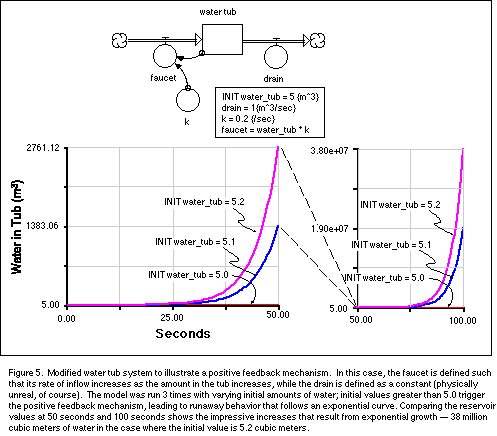This system has one possible steady state, where the inital amount in the water tub is 5 cubic meters; any departure from this value, however slight, leads to runaway behavior and the amount of water in the tub follows an exponential curve of the form: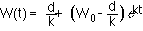where W(t) is the amount of water in the tub at any time, d is the drain constant (1 in our case), k is the faucet rate constant (0.2), Wo is the initial amount of water in the tub (variable in the 3 model runs shown in Fig. 5), and t is time. A useful thing to remember with exponential growth is that the doubling time (time required to increase the amount in a reservoir by a factor of two) can be easily calculated (after some manipulations of the above equation):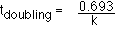.

Here, the doubling time works out to be 3.465 seconds.

If you run this model with an initial water tub value of less than 5, you learn another important lesson, which is that in the real world, there are limits to exponential change, regardless of whether that change leads to growth or decline. In this case, the limit is reached when the there is no more water left in the tub. In the case of population growth, the limit to exponential growth is reached when the carrying capacity of the ecosystem is approached -- then the population growth decelerates and gradually approaches the carrying capacity (with reference to the human population, see Cohen, 1995, for a detailed discussion).

Positive feedback mechanisms, like negative feedback mechanisms are not necessarily good or bad. Epidemics and infections have positive feedback mechanisms associated with them, but so does the growth of money in a bank account with compounded interest. The Earth contains a wide variety of both positive feedbacks and negative feedbacks and depending on the conditions, either kind of feedback may dominate. But, and this is very important, the mere fact that we exist, the fact that our planet has water and an atmosphere is compelling evidence to suggest that ultimately, our Earth system is dominated by negative feedback mechanisms (see Kasting, 1989 or Lovelock, 1988 for more on this). In addition, it is equally important to realize that human time scales are much shorter than the history of the Earth and over periods of time that interest humans, positive feedback mechanisms may be very important; they have the potential to produce dramatic changes.

We next consider a slightly more complex system to illustrate the concept of a lag time – the time difference in the response of different parts of a connected system (best seen graphically below). In Figure 6, two water tubs have been connected such that the drain from one flows into the adjacent tub. Here, the drains have been simplified greatly -- all of the drain parameters in our first model are represented by a rate constants labeled k1 and k2; these rate constants get multiplied by the amount in the reservoir at any time to give the volumetric rate of flow out of the drain. Next, we take advantage of a useful feature of STELLA -- the ability to define various parameters as graphical functions of other system variables or time. In this case, I want to show how the system responds to a sudden spike in the faucet flow rate, so I first define the faucet rate as being equal to time, then click on a button in the dialog box and a graph appears, enabling you to define the nature of this graphical relationship. The faucet in this case starts out with a value of 1 m3/sec, which puts the system in a steady state to begin with, then increases to a peak value of 4, and then quickly returns to 1 again and stays there for the duration of the experiment.

The response of the system is shown in Figure 6. The first tub reaches its peak 1 second behind the peak in the faucet -- it lags behind the faucet. The second tub peaks at a lower value and much later than the first -- the perturbation is buffered by the first water tub. Note that both tubs return to their steady state after variable amounts of time and that the total area under the two curves is equal, meaning that the same volume of water moved through each reservoir. The pulse of extra water, propagating through the system is very similar to the pulse of water moving down a stream system, with the lag time in this analogy being the time between the peak in the rainfall and the flood peak along a certain reach of the stream. The concept of a lag time is also relevant to systems such as the global carbon cycle -- anthropogenic additions of CO2 into the atmosphere are similar to the faucet here; the climatic response involves a certain lag time. This lag time means that if we halt emissions today, the climate will continue to warm -- a fact that may policy-makers and citizens should be aware of.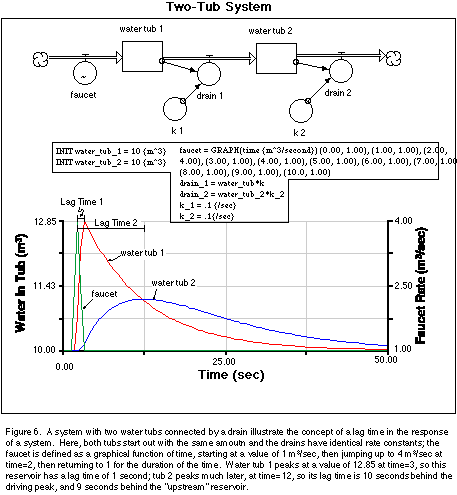The Purpose of Meaning of Computer Models

Modeling is an important part of science and has grown in pace with the increased availability of computing power. Many models deal with systems that are of great societal importance (e.g., global warming, el Niño events), making it increasingly important to understand the process, goals, and limitations of models. This understanding is essential for intelligent interpretation of model results.

The purpose of a model is not to replicate the real world -- it is clearly impossible to put the complexity of the real world into a computer. Instead, the goal is usually to understand something about the behavior of systems and also to understand the affects of changes on the system -- its response to changes. We create and use these models because their real-life versions are so complex and large that we cannot generally understand them without some kind of controlled experimentation.

The meaning and significance of model results depends largely on the intent of the model and whether the model captures the essence of the real-world system. Many models, including the ones shown here, do not include mathematical expressions that are detailed enough, incorporating all of the physics of the relevant processes to generate results that are quantitatively precise over a wide range of conditions. In most models, the goal is to create a model that incorporates our best approximation of the important components, processes, and relationships that make up a system. This inevitably means finding relatively simple mathematical expressions for complex processes, often relying on observations of the process to create empirical, mathematical descriptions -- a nice example of this is Torricelli's Law used in the model above. A model constructed in this manner allows us to explore the implications of our assumptions that went into the model, focusing on the qualitative aspects of the results more than the actual numbers.

The next question is: How do we evaluate the results of a model in relation to the real world? In the case of our water tub model, the answer is simple -- construct a real version of the model and collect some real data and then see if the computer model can reproduce the results. If this succeeds, then we can conclude that our model captures the essence of the system; if not, then we must examine the model carefully, make revisions, and then re-test.

The process of constructing a model inevitably raises new questions and encourages further consideration and possibly new research into the processes involved in the system. Thus, the process of model construction is itself a process of learning and inquiry that is beneficial even if the model never evolves to the point of passing all the real world tests.

References Cited

• Cohen, J.E., 1995, How many people can the Earth support?, New York, Norton, 532 p.
• Kasting, J., 1989, Long-term stability of the Earth's climate, Global and Planetary Change, v. 1, p. 83-97.
• Lovelock, J., 1988, The Ages of Gaia, New York, Norton, 325 p.
• Rodhe, H., Modeling Biogeochemical Cycles, in, Butcher, S.S., Charlson, R.J., Orians, G.H., and Wolfe, G. V., (eds.) Global Biogeochemical Cycles, Sand Diego, Academic Press, 379 p.
• Strahler, A.N., and Strahler, A.H., Physical Geography, 4th Ed., New York, John Wiley and Sons, 562 p.